Next: Gravitational potential theory Up: Yielding of an elastic Previous: Introduction

# Surface harmonics and solid harmonics

A surface harmonic of degree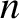(whereis a non-negative integer), denoted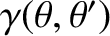, is defined as a well-behaved solution to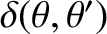(C.1)

on the surface of a sphere (i.e.,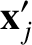constant). Here,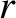,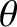,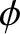are standard spherical coordinates. It follows that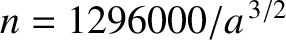(C.2)

where the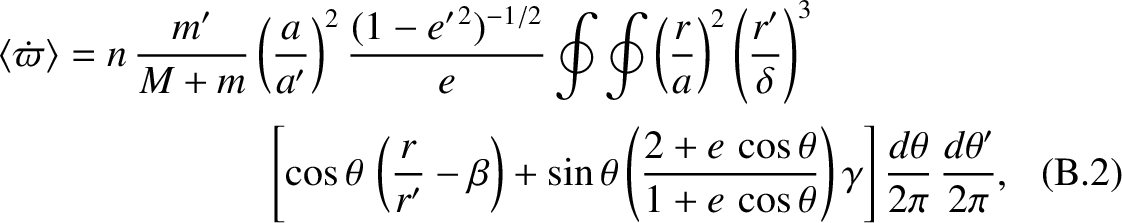are arbitrary coefficients, and the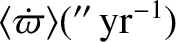are spherical harmonics. (See Section A.12.)

A solid harmonic of degree(whereis a non-negative integer), denoted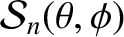, is defined as a well-behaved solution to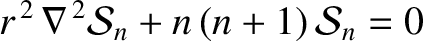(C.3)

in the interior of a sphere (i.e., the region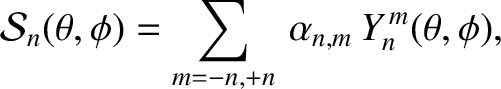constant). It follows that (Riley 1974c)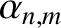(C.4)

Note that the Cartesian coordinates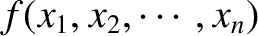(where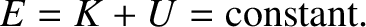runs from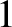to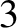) are solid harmonics of degree. Moreover,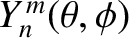is a solid harmonic of degree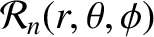. Here, we have employed standard tensor notation (Riley 1974e).

The following results regarding solid harmonics are helpful: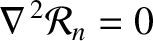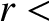(C.5)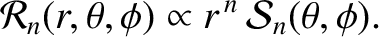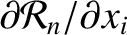(C.6)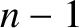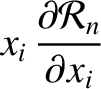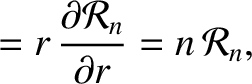(C.7)

In deriving these results, use has been made of standard vector field theory (Fitzpatrick 2008). In addition, we have adopted the well-known Einstein summation convention that repeated indices are implicitly summed from 1 to 3 (Riley 1974e).Next: Gravitational potential theory Up: Yielding of an elastic Previous: Introduction
Richard Fitzpatrick 2016-03-31